# MSBSHSE Class 8 Science Chapter 4 Current Electricity and Magnetism Textbook Solutions

In MSBSHSE Class 8 Science Chapter 4 Current Electricity and Magnetism, students learn about electricity and magnetic properties of objects. Here, we bring you the important questions and solutions of the chapter that will help students to get a thorough understanding of all the key concepts and master it properly.

Our subject matter experts have covered the explanations of important questions and solutions of MSBSHSE Class 8 Science of all the chapters. We hope that students can benefit from it and discover new concepts, while prepping up for the MSBSHSE Class 8 2020 exam. All the important questions of the MSBSHSE Class 8 Science Solutions for Chapter 4 Current Electricity and Magnetism have been extensively covered to help students to grasp an in-depth knowledge of important concepts in a lucid manner.

In this article, we have thoroughly covered all the exercise questions and added a few additional questions along with solutions for Chapter 4 from the MSBSHSE Class 8 Science Syllabus. Students can start practising from these questions while studying this chapter and build a rock-solid foundation of concepts that are discussed in it, revise those concepts before their exams and score well in this subject.

## Maharashtra Board Class 8 Science Chapter 4: BYJU’S Important Questions & Answers

### MSBSHSE Class 8 Science Chapter 4 Textbook Exercise Questions

Question 1: Write proper words from the following group of words in the blanks.

(magnetism, 4.5V, 3.0V, gravitational attraction, potential difference, potential, higher, lower, 0V)

a.Water in the waterfall flows from a higher level to the lower level because of ____________.

b. In an electric circuit, electrons flow from a point of ________ potential to the point of ____________ potential.

c. The difference between the electrostatic potential of the positive end and the negative end of an electric cell is the __________ of the cell.

d. Three electric cells of potential difference 1.5V each have been connected as a battery. The potential differences of the battery will be ________ V.

e. An electric current flowing in a wire creates __________ around the wire.

Answer: a. Water in the waterfall flows from a higher level to the lower level because of potential differences.

b. In an electric circuit, electrons flow from a point of higher potential to the point of lower potential.

c. The differences between the electrostatic potential of the positive end and the negative end of an electric cell is the potential difference of the cell.

d. Three electric cells of potential difference 1.5 V each have been connected as a battery. The potential differences of the battery will be 1.5 + 1.5 + 1.5 = 4.5V.

e. An electric current flowing in a wire creates a magnetic field around the wire.

Question 2: A battery is to be formed by joining 3 dry cells with connecting wires. Show how you will connect the wires by drawing a diagram.

Answer: Given below is the diagram used to show the connection by joining 3 dry cells with connecting wires: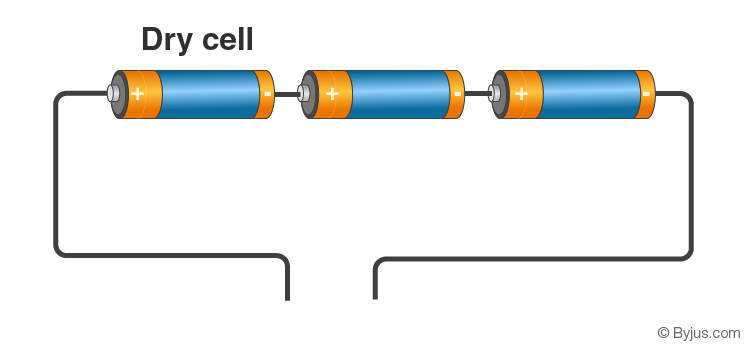or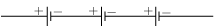Question 3: In an electric circuit, a battery and a bulb have been connected and the battery consists of two or three cells of equal potential difference. If the bulb is not glowing, then which tests will you perform in order to find out the reason for the bulb not glowing?

Answer: We need to perform the following test to detect the reason why the bulb is not glowing:

1. First, we need to check how the battery terminals are connected to each other. It should be ensured that the positive terminal of a battery is connected to the negative terminal of another battery. If all the batteries are linked or connected in this fashion and even then the bulb is not glowing, then we need to check an alternate option.

2. Secondly, we need to check if there are any broken wires in the circuit. By ensuring that the wires that are used for linking or connecting the different electrical components are not broken in between it helps in detecting that the circuit is closed. Further, this also helps to ensure that the wires are not broken somewhere in between because of which the bulb is not glowing. If this doesn’t work, we need to move to the next test.

3. Finally, we need to check how the connecting wires are plugged into the bulb. This will ensure that the bulb is properly connected to the batteries as shown in the diagram below. Even then, if the bulb does not glow, then we need to replace the batteries of the bulb with a new set.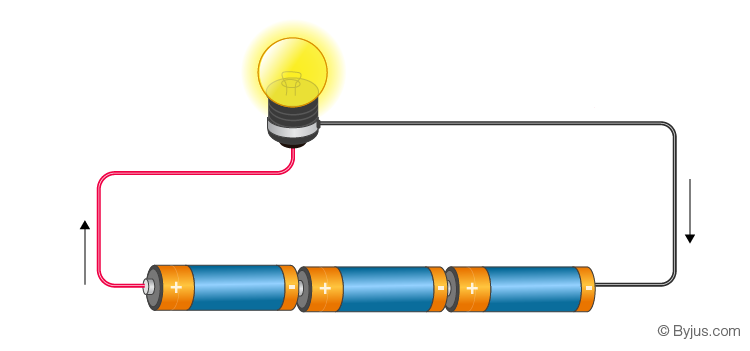Question 4: Electric cells having 2V potential difference each have been connected in the form of a battery. What will be the total potential difference of the battery in both cases ?

(i)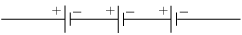(ii)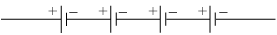Answer: (i) Total potential difference of electric cells having 2V each = 2 + 2 + 2 = 6V

(ii) Total potential difference of electric cells having 2V each = 2 + 2 + 2 + 2 = 8V

Question 5: Describe the construction, working and usefulness of a dry cell, with the help of a diagram.

Answer: Construction of dry cell: A dry cell consists of the following components –

1. Outer metal cover: The outer metal cover is made up of Zinc metal. It is white in appearance and works as a negative terminal of the cell.

2. Electrolyte: There is the electrolyte filled between the two layers inside the Zinc metal. Electrolyte is a wet pulp which is a combination of Ammonium chloride (NH4Cl) and Zinc chloride (ZnCl2). It is the carrier of electric charge as it consists of both positively charged and negatively charged ions.

3. Metal rod: At the centre of the cell, there is a graphite rod which is surrounded by a paste of Manganese dioxide (MnO2). It is considered the positive terminal of the cell.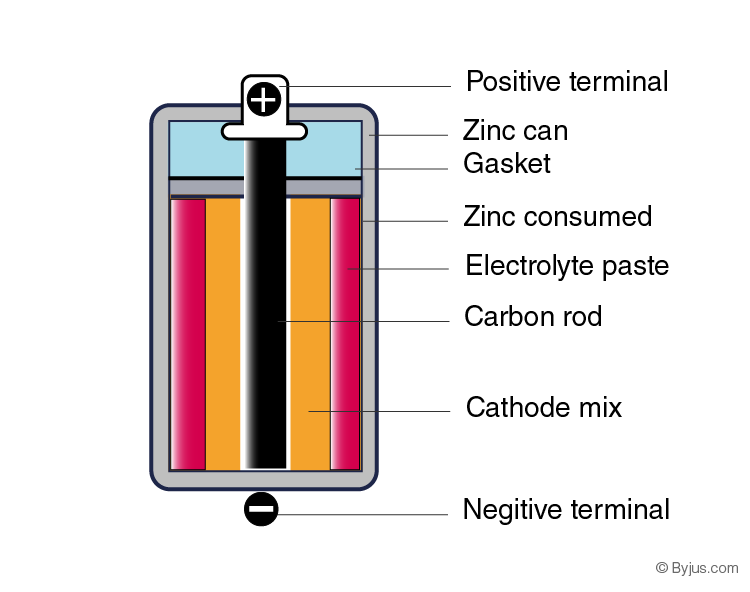4. Working of a dry cell: The chemical reactions take place between the zinc container, electrolyte and graphite rod. Due to this reason, electric charge is produced or generated on the two terminals – positive and negative of the cell and electric current gradually flows into the circuit.

5. Usefulness of a dry cell: A dry cell is usually portable and easy to use. The longevity of a dry cell is more than those cells which use liquid electrolyte. Dry cells can also be used in T.V., torch, toys or Air Conditioner remote controls, etc.

Question 6: Describe the construction and working of an electric bell with the help of a diagram.

Answer: Construction of an electric bell: The components of electric bell are given below –

• Electromagnet: A copper wire is wrapped around an iron piece that works as a magnet when the electric current flows through it.
• The hammer is connected with the contact screw through an iron strip.
• A metal gong.
• A switch or key.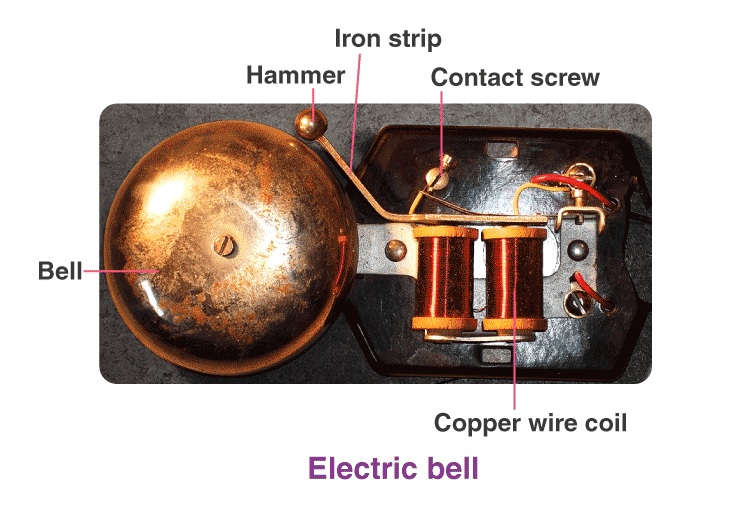Working of an electric bell

When the switch is pressed ‘ON’, the screw is connected with the iron strip, then electric current flows through the copper wire and gets magnetised due to electromagnetism. This electromagnet or magnetised copper wire attracts the iron strip towards it and lets the striker hit the gong which produces sound.

As soon as the hammer hits the gong, the screw loses its touch with the iron strip and the electric current stops in the circuit. Now, the electromagnet tends to lose its magnetic power and the iron strip moves backward which then comes in contact with the contact screw. Therefore, the electric current is thereafter restored in the circuit and again the hammer hits the gong as per the above mentioned process. This action is an ongoing process and it repeats itself and the bell rings to produce sound.

To explore in detail about the working of an Electric Bell, check at the linked article.

### MSBSHSE Class 8 Science Chapter 4 Additional Questions

Question 1: What are the constituents of an atom?

Answer: An atom has the same number of positively charged protons and negatively charged electrons in it.

Question 2: Describe current electricity.

Answer: An enormous current flows when lightning strikes from a cloud to the ground, while we feel the sensation due to the microscopically small current flowing to the brain. We are all aware of the current flowing through electric bulbs, wires and electrical equipment in the house. In the electric cells of a car battery or a radio, a current is produced by the flow of both positively and negatively charged particles.

Question 3: Define electrons.

Answer: Moving charges transfer from one object to another as these are negatively charged. Such moving negatively charged particles are called electrons.

Question 4: What is electrostatic potential?

Answer: We are also aware that water or a liquid flows from a higher level to a lower level due to the gravitational force on earth. Likewise, heat always flows from a body at higher temperature to a body at lower temperature. In similar fashion, there is a tendency of the positive charge to flow from a point of higher electric level to a point of lower electric level. This electric level deciding the direction of flow of electric charges is known as electrostatic potential.

Question 5: What is a potential difference?

Answer: Electric potential difference or electric tension is the difference in electric potential between two points, i.e. the temperature difference of hot and cold bodies or that similar to the height of a waterfall, etc.

Question 6: How is the electric current measured?

Answer: Electric current is produced due to the flow of both positively and negatively charged particles. When it flows through a wire in one second, it can be called unit current. The SI unit

of electric current is Coulomb per second or Ampere. Electric current is a scalar quantity.

1 Ampere = 1A = 1 Coulomb/1 second = 1 C/s.

Question 7: Define an electric cell. Give examples.

Answer: A general device that is required to produce a uniform flow of charges in an electric circuit. Such a general device is referred to as an electric cell. These are used in ships, wrist watches, submarines, etc.

Question 8: What is the main function of electric cells?

Answer: The main function of different electric cells is to maintain a constant potential difference between its two terminals. The electric cells usually work on the electric charges to maintain a constant potential difference between the terminals.

Question 9: What is a dry cell? Give examples.

Answer: A dry cell is a type of electric battery or unit which is mostly used in portable electrical devices. Dry cells are used in our TV or AC remote controls, radio sets, wall clocks and torches, etc.

Question 10: What is an electrolyte?

Answer: An electrolyte is filled between the two layers of Zinc (Zn) metal and graphite rod of a dry cell. It contains both negatively charged and positively charged ions in it. These are the carriers of electricity.

Question 11: What are lead-acid cells used for?

Answer: Lead-acid cells are mostly used in vehicles such as lorries, cars, motorbikes and generators such as Uninterrupted Power Supplies (UPS).

Question 12: What is an electric circuit?

Answer: When an electric bulb, a cell holder and a plug key are connected through connecting wires and a dry cell is fitted into the holder, then the bulb lights up by closing the plug key. This implies that the bulb lights up due to the flow of the current through the circuit. When we remove the cell, the electric current flowing through the circuit suddenly stops and the light in the bulb ceases to glow. This type of connection of electrical components is known as an electric circuit.

Question 13: What are connecting cells?

Answer: When two or more cells are connected to an electric circuit in a series, this obtains more potential difference than that of a single cell. This connection of cells is known as a battery of cells. Connecting cells in a series increases the voltage of the circuit but doesn’t make the cells last longer.

Question 14: Where are Lithium ion cells used?

Answer: Lithium ion cells are mostly used in modern equipment such as smartphones, laptops, etc. These cells are the ones which can be recharged. Additional electrical energy can also be stored in these cells in comparison to those of Nicd cells.

Question 15: What is the magnetic effect of electric current?

Answer: The magnetic effect of electric current is referred to as electromagnetic effect. It is observed that when a magnetic bar is brought near the magnetic needle, the needle gets deflected and changes its direction because of the flow of electricity. This shows that electric current flows in a wire and a magnetic effect is produced.

We hope that the above mentioned solutions of “MSBSHSE Class 8 Science Chapter 4 Current Electricity and Magnetism” will help students to build a strong and solid foundation of the different concepts mentioned in the chapter.

## Frequently Asked Questions on Maharashtra State Board Solutions for Class 8 Science Chapter 4 Current Electricity and Magnetism

### Are these Maharashtra State Board Class 8 Science Chapter 4 Solutions useful to prepare for the exams?

Yes, these Maharashtra Board Class 8 Science Chapter 4 Solutions are very helpful. Students are also recommended to practise these solutions after revising the subjects because they set the basis for the questions that could get asked often in the board exams.